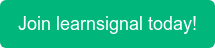Volatility
is a statistical measure of a security's or market index's return dispersion. The more the volatility, the riskier the security is in most circumstances. A "volatile" market, for example, is one in which the stock market rises and falls by more than 1% over a long time.

There are several ways to measure volatility, including beta coefficients, option pricing models, and standard deviations of returns.

Example of Volatility:

The formula for daily volatility is computed by finding out the square root of a daily stock price variance.

Daily Volatility Formula is represented as,

Daily Volatility formula = √Variance

Further, the annualised volatility formula is calculated by multiplying the daily volatility by a square root of 252.

Why is Volatility important?

Volatility is a measure of risk, while volatility measures variability. It assists investors in assessing the risk they take when purchasing a specific asset and determining if the purchase will be worthwhile.

Topics: ACCA, CIMA, CPD, AAT, FRM•### Central Counterparties

•### Country Risk

•### Convexity Formula

•### Clean and Dirty Price

•### Basic Indicator Approach

•### ACCA Exam Fees 2022: Everything You Need to Know

•### ACCA Exam Dates for 2022

•### How Difficult is Passing ACCA? An Honest Review

•### 7 of the Best YouTube Channels for Accounting Students

•